Open in App
Not now

# Fluid Friction

• Last Updated : 18 Nov, 2021

Fluid friction occurs between fluid layers that are moving relative to each other. This internal resistance to flow is named viscosity or viscous drag or fluid friction. In normal terms, the viscosity of a fluid is described as its “thickness” of the fluid. Thus, water is “thin”, having a lower viscosity, while honey is “thick”, having a higher viscosity. The less viscous the fluid, the greater its ease of deformation or movement.

All real fluids (except superfluids) offer some resistance to shearing and therefore are viscous. Although the term “fluid” includes both the liquid and gas phases, in common usage, “fluid” is often used synonymously with “liquid”. Liquids and gases are called fluids because they can be made to flow or move. In any fluid, the molecules themselves are in constant, random motion, colliding with each other and with the walls of any container.

The movement of molecules is governed by two types of forces which are present between the molecules of the substance itself and between the substance and the other material in contact.

The following are the two types of forces between the molecules:

1. Cohesive forces: Cohesive forces are attractive forces that exist between molecules of the same substance. So, cohesive forces are the main cause of viscosity.

2. Adhesive forces: These are attractive forces between unlike molecules.

An object travels in a fluid it loses its energy in order to overcome the fluid Friction. The shape of an object plays an important role in increasing or decreasing the fluid friction when it travels along with it.

• Ships and boats have a particular shape that allows them to resist fluid Friction.
• Birds and airplanes have a specific shape that allows them to fly in the air.
• Fishes also have a specific shape such as a tail and fins that allow them to swim continuously in water and even prevent the loss of energy due to fluid Friction.
• The designs of vehicles are made in such a way so that they encounter the least fluid friction while travelling to ensure proper usage and movement of them.
• In order to avoid fluid friction the swimsuits are designed accordingly for a smoother flow.

### Laws Of Fluid Friction

The laws of fluid friction are:

First law: The fluid friction increases with an increase in the area of contact between the surface and the fluid.

Second law: The fluid friction increases with an increase in the velocity gradient of the substance.

Third law: The fluid having a higher coefficient of fluid friction or eta (η) has a higher value of fluid frictional force.

The rules hold differently for different lubricants. It is indirectly proportional to the temperature of the lubricants. The formula for the fluid friction can be derived by the following experiment: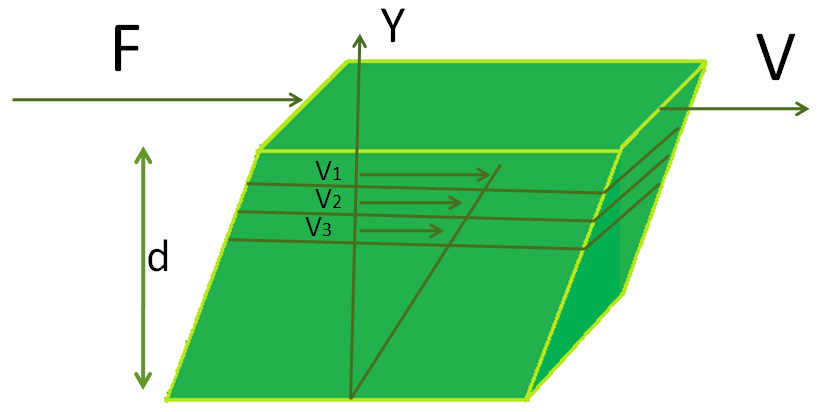In the given experiment it can be shown that the force Ϝ necessary to move the top plate in the figure is directly proportional to the area Α, the difference of speed between the layers ∆ν and inversely proportional to the spacing of the plates ∆y.

That is: Ϝ∽Α and Ϝ∽∆ν and Ϝ∽1∕∆y

This gives the equation Ϝ=η Α ∆ν∕∆y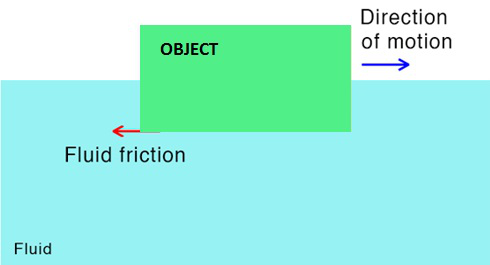Fluid Friction

### Factors Affecting Fluid Friction

The amount of Frictional force by a fluid on an object depends upon the following:

1. Nature of fluid

The thinner the fluid, the lesser the fluid friction will be, and vice versa. The different types of fluid offer different extent of resistances or the viscous drag of the object flowing through it. For example, an object moving in honey experiences a much larger viscous force than moving in plain water as the density of honey is much larger than the density of water and hence it offers greater resistance to the flow of the object.

2. The viscosity of fluid

The viscosity of fluid plays a major role in determining the velocity of the object which flows through it. The more viscous is the fluid the denser it is and hence the movement of any material inside it is restricted or experiences resistance to its motion. The denser is the liquid the more viscous drag it creates.

3. The shape of the body

Other figures feel more drag than the body with a streamlined shape which begins and ends in points like the shape of a fish. The shapes of vehicles are designed to reduce the surface of contact during motion. It plays a major role in the smoother flow be it in liquid or in gases. The shape of the body has to be in accordance with the medium in which it is flowing or moving or else it experiences a major resistance to its movement which makes the task very time-consuming. Hence, to reduce this most of the bodies have a streamlined shape. This ensures that at the start and at the end the surface of contact is minimum and hence the fluid friction.

4. The temperature

The fluid friction of all gases increases with an increase in temperature since the fluid friction of a gas is directly proportional to the square root of the absolute temperature. (This result is obtained from the kinetic theory of gases).

The fluid friction of liquids and other substances also increases proportionally with the increase in temperature.

5. The surface area of the body

The more the surface of the body more is the fluid friction. For example A horizontal moving body can cut through the liquid more easily than a body moving vertically. For a body with a large surface area, the fluid friction increases accordingly and hence its movement or flow becomes difficult.

6. Speed of the body

Friction and speed are directly proportional to each other. On increasing the speed the fluid friction or drag also increases proportionally. For example: An airplane flying at the highest speed of 1000km/hr. Will face a greater frictional force or drag of air than another similar airplane which is flying at a speed of 600km/hr. Or a high-speed boat experiences lesser fluid friction than a slow-moving fish.

7. The pressure on the body

The fluid friction increases proportionally with the increase in the pressure on the body. With the increase in pressure, it becomes difficult for the molecules to travel from one place to another place and also over the layers. Hence, there is a huge amount of resistance to the flow of the fluids.

### What is Viscous Drag?

A resistance force exerted on a moving object by the fluid is called Viscous drag. The speed at which the viscous drag of an object falling in a viscous fluid is equal to the other forces acting on the object (such as gravity) so that the acceleration of the object is zero. Everyone has seen fluids flow. From pouring a glass of water to watching maple syrup creep towards the edge of a stack of pancakes. Viscosity is the intrinsic resistance of a fluid to flow under an applied force.

Let’s go through a more technical explanation of viscous drag and viscosity by the following diagram: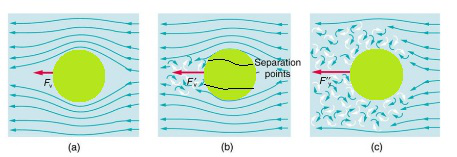Let’s go back to the maple syrup again and pretend we are freezing it into thin slabs that are stacked one on top of another.

Diagram 1: Shows these layers and a force, F, applied to the top slab of frozen syrup.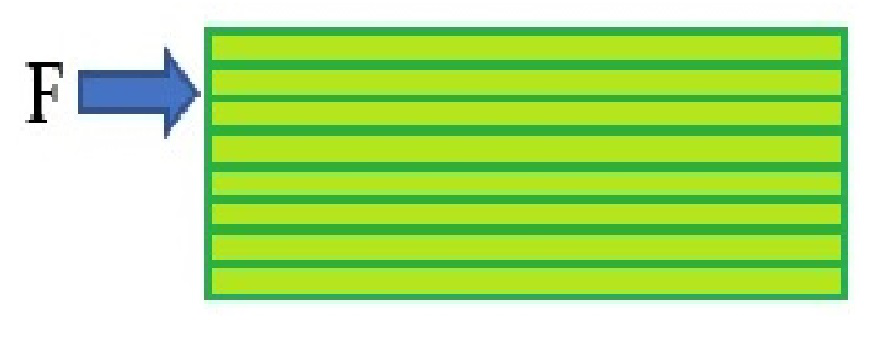Since the applied force is acting on the top layer, we would expect the top layer to be pushed out right?

The friction between the top layer and the second layer would drag the second layer along. This will continue down through the layers of slabs with each towards the bottom moving less than the layer above it.

Diagram 2: Shows this effect in action.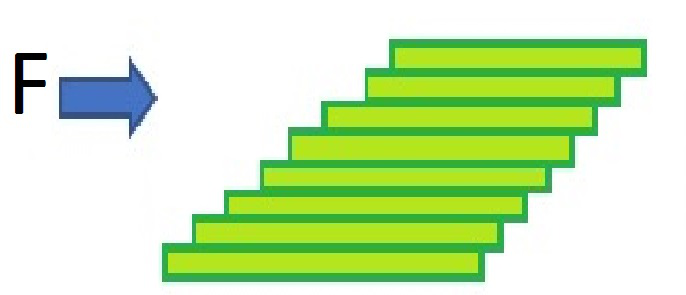### Sample Problems Based On Fluid Friction

Question 1: Boats and airplanes are made in streamlined shape. Why?

A shape which is wide in middle and tapered at ends is called a streamlined shape. Such a shape reduces drag or fluid friction.

Hence, a streamlined body can easily move through a fluid. Birds and fish are naturally endowed with streamlined body. Boats and airplanes are also made streamlined so that they can easily move through air or water.

Question 2: Why are the bodies of boats, planes, cars, etc. streamlined?

For objects that move in fluids such as boats, planes, cars, etc.

The shape of their body is streamlined in order to reduce the friction between the bodies of the objects as the fluid.

Question 3: How can we land smoothly using a parachute with the help of Viscous drag?

When a parachute is just opened, the velocity is down and the acceleration is up. Your speed decreases as a result, which is the whole point behind the parachute.

Speed decreases, so drag decreases. Drag decreases, so the net force decreases.

Question 4: What are the advantages of a streamlined body?

Streamlining your body reduces the friction of movement to a minimum thus decreasing overall drag.

For fishes having a streamlined body that is smooth helps save energy it would otherwise have to expand swimming.

Question 5: What is laminar flow?

Laminar flow, type of fluid (gas or liquid) flow in which the fluid travels smoothly or in regular paths, in contrast to turbulent flow, in which the fluid undergoes irregular Fluctuations and mixing.

Question 6: Which of the following statements is incorrect?

(a) Friction acts on a ball rolling along the ground

(b) Friction acts on a boat moving on water

(c) Friction acts on a bicycle moving on a smooth road

(d) Friction does not act on a ball moving through air

(d) Friction will act in case of ball moving through air always.

Question 7: What is the velocity gradient if frictional force is given to be 6N and eta(????) is given as 0.25 and the area of cross-section is 100m2.

Solution:Question 8: Determine the force of friction in fluids if the coefficient of fluid friction is 0.25, velocity with which the fluid is flowing is 2m/s starting from the rest and the cross-sectional area is 50m2.

Solution:Question 9: Determine the coefficient of fluid friction if F is given 3N and the area of cross section is given 10m2 also the velocity gradient given is 0.3/s.

Solution:

We know,Coefficient of fluid friction is 1.

My Personal Notes arrow_drop_up